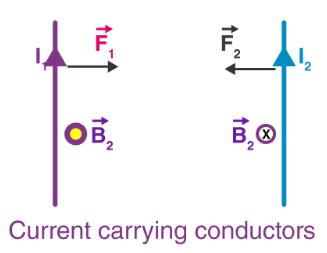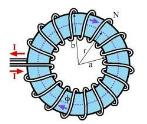Courses

# Test: The Solenoid And Toroid

## 10 Questions MCQ Test Physics Class 12 | Test: The Solenoid And Toroid

Description
This mock test of Test: The Solenoid And Toroid for JEE helps you for every JEE entrance exam. This contains 10 Multiple Choice Questions for JEE Test: The Solenoid And Toroid (mcq) to study with solutions a complete question bank. The solved questions answers in this Test: The Solenoid And Toroid quiz give you a good mix of easy questions and tough questions. JEE students definitely take this Test: The Solenoid And Toroid exercise for a better result in the exam. You can find other Test: The Solenoid And Toroid extra questions, long questions & short questions for JEE on EduRev as well by searching above.
QUESTION: 1

### The magnetic field inside a toroidal solenoid of radius R is B. If the current through it is doubled and its radius is also doubled keeping the number of turns per unit length the same; magnetic field produced by it will be:

Solution:

Magnetic field is given by μ0nlμ0nl. Here / is doubled and n is unchanged.

QUESTION: 2

### The force between two current carrying conductors is due to which of the following

Solution:

we can say that any two current carrying conductors when placed near each other, will exert a magnetic force on each other.Consider the system shown in the figure above. Here, we have two parallel current carrying conductors, separated by a distance ‘d’, such that one of the conductors is carrying a current I1 and the other is carrying I2, as shown in the figure. From the knowledge gained before, we can say that the conductor 2 experiences the same magnetic field at every point along its length due to the conductor 1. The direction of magnetic force is indicated in the figure and is found using the right-hand thumb rule. The direction of the magnetic field, as we can see, is downwards due to the first conductor.

QUESTION: 3

### Which of the following laws give the direction of induced e.m.f

Solution:

Lenz’s law is used for determining the direction of induced current.

Lenz’s law of electromagnetic induction states that the direction of induced current in a given magnetic field is such that it opposes the induced change by changing the magnetic field.

Following is the formula of Lenz’s law:

ϵ=−N (∂ϕB/∂t)

Where,

• ε is the induced emf

• ∂ΦB is the change in magnetic flux

• N is the number of turns in the coil

Lenz’s law finds application in electromagnetic braking and in electric generators

QUESTION: 4

What is the magnetic field in the empty space enclosed by the toroidal solenoid of radius R?

Solution:

The magnetic field is only confined inside the body of a toroid in the form of concentric magnetic lines of force. For any point inside the empty space surrounded by toroid and outside the toroid, the magnetic field B is zero because the net current enclosed in these spaces is zero. Thus, the magnetic moment of the toroid is zero.

QUESTION: 5

The magnetic field inside a toroid of radius R is B. If the current through it is doubled and the radius increased four times keeping the number of turns per unit length same, then the magnetic field produced by it will be

Solution:

In the 1st case,
B1= μ0Ni/2πr
In the 2nd case,
B2= μ0N2i/2π4r
Comparing both of them,
μ0Ni/2πr=μ0N2i/2π4r
B1=B2/2
B2=2B1

QUESTION: 6

A solenoid coil of 300 turns /m is carrying a current of 5 A. Calculate the magnitude of magnetic intensity inside the solenoid.​

Solution:

We know, Magnetic field of solenoid,
= μonI
=4πx10-7x300x5
=4πx15x10-5
=6πx10-9
=18.89x10-9
≈1.9x10-3T

QUESTION: 7

Magnetic field due to a current carrying toroidal solenoid is independent of:

Solution:

For a toroidal solenoid, B=μ0nI
Where, B is the magnetic field, μ0 is the permeability of free space, n is the number of coils in the solenoid per length (like 2 turns per millimetre, let's say), and I is the electric current.
i.e. It does not depend upon its radius.

QUESTION: 8

In a current carrying conductor varying electric field generates

Solution:

In a current-carrying conductor, by varying the electric current, the magnetic field is generated. The direction of the magnetic field generated will be perpendicular to the conductor.
The direction of the magnetic field can be determined as wrapping the right-hand fingers around the wire and the direction of the current is indicated by the thumb.
Ampere’s law is used for determining the magnetic field of a long conductor:
B=μ0I/2πr
Where,

• I is the current in Amperes

• r is the radial distance in metres

• B is the magnetic field in Tesla

QUESTION: 9

The magnetic field at the ends of a solenoid is

Solution:

It is true only for an infinitely long solenoid.
Magnetic field at any point is the summation of magnetic fields produced by each turn of the wire of the solenoid.
Now imagine a solenoid in front of you stretching to infinity on both the sides, say from left to right. An infinitely long solenoid has no ends and the field everywhere inside it is the same and let its intensity be E. Now cut the solenoid at a point in front of you and throw (!) the half away. You now have in front of you, an end of the remaining half. The field at this end is now due to half the total turns and hence it is E/2.

QUESTION: 10

Magnetic field outside the toroid is

Solution:

A toroid is shaped like a solenoid bent into a circular shape such as to close itself into a loop-like structure. The toroid is a hollow circular ring, as can be seen in the image shown below, with a large number of turns of enamelled wire, closely wound with negligible spacing between any two turns.The magnetic field inside and outside the toroid is zero. The magnetic field inside the toroid, along the circular turn, is constant in magnitude and its direction inside the toroid is clockwise as per the right-hand thumb rule for circular loops.
The magnetic field due to a toroid can be given as,
B= μ0NI/2πr
Where N is the number of turns of the toroid coil, I is the amount of current flowing and r is the radius of the toroid.